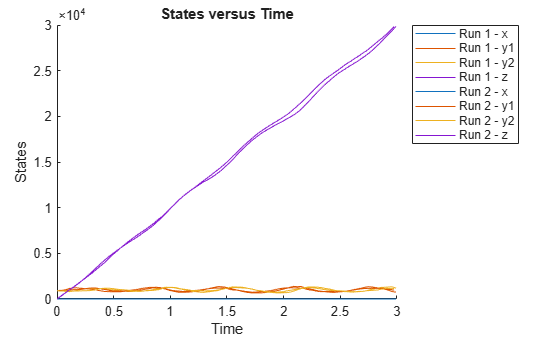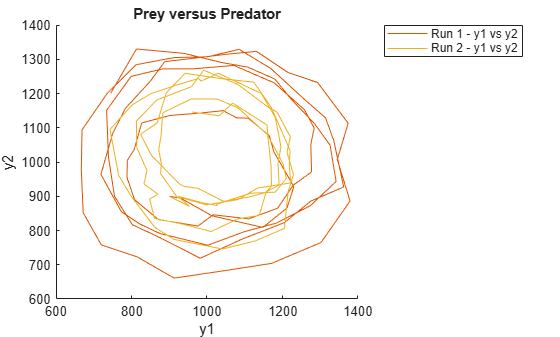Main Content

# sbioplot

Plot simulation results in one figure

## Syntax

``sbioplot(sd)``
``sbioplot(sd,fcnHandle,xArgs,yArgs,Name,Value)``

## Description

example

````sbioplot(sd)` plots each simulation run from `sd`, a `SimData` object or array of objects, in the same figure. The plot is a time plot of each state in `sd`. The figure also shows a hierarchical display of all the runs as different nodes in a tree, and you can select which run to display.```

example

````sbioplot(sd,fcnHandle,xArgs,yArgs,Name,Value)` plots simulation results by calling the function handle `fcnHandle` with inputs `sd`, `xArgs`, and `yArgs`, and uses additional options specified by one or more name-value pair arguments. For example, you can specify the x-label and y-label of the plot. `xArgs` and `yArgs` must be cell arrays or string vectors of the names of the states to plot.```

## Examples

collapse all

Plot the prey versus predator data from the stochastically simulated lotka model by using a custom function (`plotXY`).

Load the model. Set the solver type to SSA to perform stochastic simulations, and set the stop time to 3.

```sbioloadproject lotka; cs = getconfigset(m1); cs.SolverType = 'SSA'; cs.StopTime = 3; rng('default') % For reproducibility```

Set the number of runs and use `sbioensemblerun` for simulation.

```numRuns = 2; sd = sbioensemblerun(m1,numRuns);```

Plot the simulation data. By default, `sbioplot` shows the time plot of each species for each run.

`sbioplot(sd); `Plot selected states against each other; in this case, plot the prey population versus the predator population. Use the function `plotXY` (shown at the end of this example) to plot the simulated y1 (prey) data versus the y2 (predator) data. Specify the function as a function handle.

If you use the live script file for this example, the `plotXY` function is already included at the end of the file. Otherwise, you must define the `plotXY` function at the end of your .m or .mlx file or add it as a file on the MATLAB path.

`sbioplot(sd,@plotXY,{'y1'},{'y2'},'xlabel','y1','ylabel','y2','title','Prey versus Predator');`Define plotXY Function

sbioplot accepts a function handle for a function with the signature:

`function [handles,names] = functionName(sd,xArgs,yArgs)`.

The `plotXY` function plots two selected states against each other. The first input `sd` is the simulation data (SimBiology `SimData` object or vector of objects). In this particular example, xArgs is a cell array containing the name of the species to be plotted on the x-axis, and yArgs is a cell array containing the name of the second species to be plotted on the y-axis. However, you can use the inputs xArgs and yArgs in any way in your custom plotting function. The function returns `handles`, an array of function handles to the line plots, and `names`, a cell array of character vectors shown on the nodes that are children of a Run node in a hierarchical display.

```function [handles,names] = plotXY(sd,xArgs,yArgs) % Select simulation data for each state from each run. xData1 = selectbyname(sd(1),xArgs); xData2 = selectbyname(sd(2),xArgs); yData1 = selectbyname(sd(1),yArgs); yData2 = selectbyname(sd(2),yArgs); % Plot the species against each other. fH1 = plot(xData1.Data,yData1.Data); fH2 = plot(xData2.Data,yData2.Data); % The first output, handles, is a two-dimensional array of handles of the line plots. It must be of size M x N, % where M is the number of line plots for each run and N is the number of runs. handles = [fH1,fH2]; % The second output, names, must be a one-dimensional cell array of character vectors. % Its length must be equal to the number of rows in handles, and the texts are displayed on the % nodes that are children of a Run node. names = {'y1 vs y2'}; end```

## Input Arguments

collapse all

Simulation results, specified as a `SimData` object or vector of `SimData` objects.

This argument corresponds to the first input of the function referenced by `fcnHandle`.

Example: `simdata`

Function to generate line plots, specified as a function handle. For an example of a custom function to plot selected species from simulation data, see Plot Selected States from Simulation Data.

The function must have the signature:

```function [handles,names] = functionName(sd,xArgs,yArgs)```.

The inputs `sd`, `xArgs`, and `yArgs` are the same inputs that you pass in when you call `sbioplot`.

The first output `handles` is a two-dimensional array of handles of the line plots generated by the function. Its size must be P-by-R, where P is the number of line plots, and R is the number of runs.

The second output `names` is a one-dimensional cell array of character vectors containing the names to be displayed on the nodes that are children of a Run node in a hierarchical display. The length of `names` must be equal to the number of rows in `handles`.

Example: `@plotXY`

Data Types: `function_handle`

State names to plot, specified as a string vector or cell array of character vectors. For instance, you can use `xArgs` to represent the states to be plotted on the x-axis of your custom plot.

This argument corresponds to the second input of the function referenced by `fcnHandle`.

Example: `{'y1'}`

Data Types: `cell`

State names to plot, specified as a string vector or cell array of character vectors. For instance, you can use `yArgs` to represent the states to be plotted on the y-axis of your custom plot.

This argument corresponds to the third input of the function referenced by `fcnHandle`.

Example: `{'y2','z'}`

Data Types: `cell`

### Name-Value Pair Arguments

Specify optional comma-separated pairs of `Name,Value` arguments. `Name` is the argument name and `Value` is the corresponding value. `Name` must appear inside quotes. You can specify several name and value pair arguments in any order as `Name1,Value1,...,NameN,ValueN`.

Example: `'title','Species X versus Species Y'` specifies the axes title of the plot.

Axes title, specified as the comma-separated pair consisting of `'title'` and character vector or string.

Example: ```'title','Prey versus Predator'```

Data Types: `char` | `string`

Label for the x-axis of the plot, specified as the comma-separated pair consisting of `'xlabel'` and a character vector or string.

Example: `'xlabel','y1'`

Data Types: `char` | `string`

Label for the y-axis of the plot, specified as the comma-separated pair consisting of `'ylabel'` and a character vector or string.

Example: `'ylabel','y2'`

Data Types: `char` | `string`

## Compatibility Considerations

expand all

Behavior changed in R2020a

## See Also

Introduced in R2008a

## Support평가판 신청